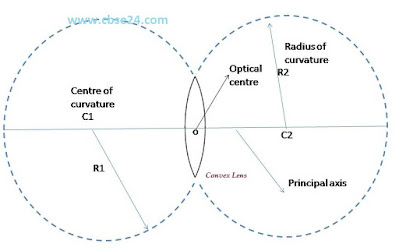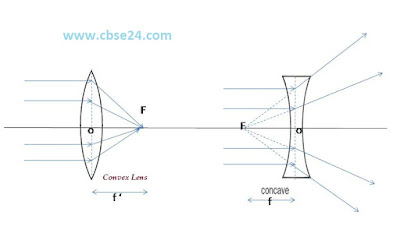# Spherical Lenses Basic class 10 |cbse24

Most of us are familiar with lenses. As magnifying glasses, lenses have been in use for centuries. Lenses used in spectacles help us to read with comfort, varies optical instruments like camera, projector, microscope, telescope etc., cannot function without lenses.(A lens is a piece of a refracting medium bounded by two surface at least one of which is a curved surfaced)

Lenses can be divided into two categories

1:Convex or converging lenses

2:Concave or diverging lenses

### Definitions in connection with spherical lenses:-

1: Center of curvature(C):-The Center of curvature of the surface of a lens is the centre of the sphere of which it forms a part. Because a lens has two surfaces, so it has two centres of curvature.

2:Radius of curvature(R):-The radius of curvature of the surface of a lens is the radius of the sphere of which the surface forms a part.

3:-Principal axis(C1C2):-It is the line passing through the two centres of curvature of the lens.4:Optical centre:-if a ray of light is incident on a lens such that after refraction through the lens the emergent ray is called to the incident ray, then the point at which the refraction ray intersects the principal axis is called the optical centre of the lens.5:Principal foci and focal length:

(i) First principal focus:-It is a fixed point on the principal axis, such that rays starting from this point(in convex lens) or appearing to go towards this point(in concave lens) after refraction of the lens become parallel to the principal axis.

It is represented by F1or F'. The distance between the first principal focus and the optical centre is called the first focal length. It is denoted by f1 or f'.(ii)Second Principal focus: It is fixed point on the principal axis such that the light rays incident parallel to the principal axis, after refraction through the lens, either converge to the point (in convex) or appear to diverge from this point (in concave lens). The plane passing through this point and perpendicular to the principal axis is called the second focal plane.Note:-

1:In both first (f ') and second (F) of convex lenses is real

2:In both first(f ') and second (F) foci of concave lens is virtual

3:If the medium on both sides of a lens is the same, then the numerical value of the first and second focal lengths are equal, thus f=f '

Aperture:-It is the diameter of the circular boundary of the lens.*